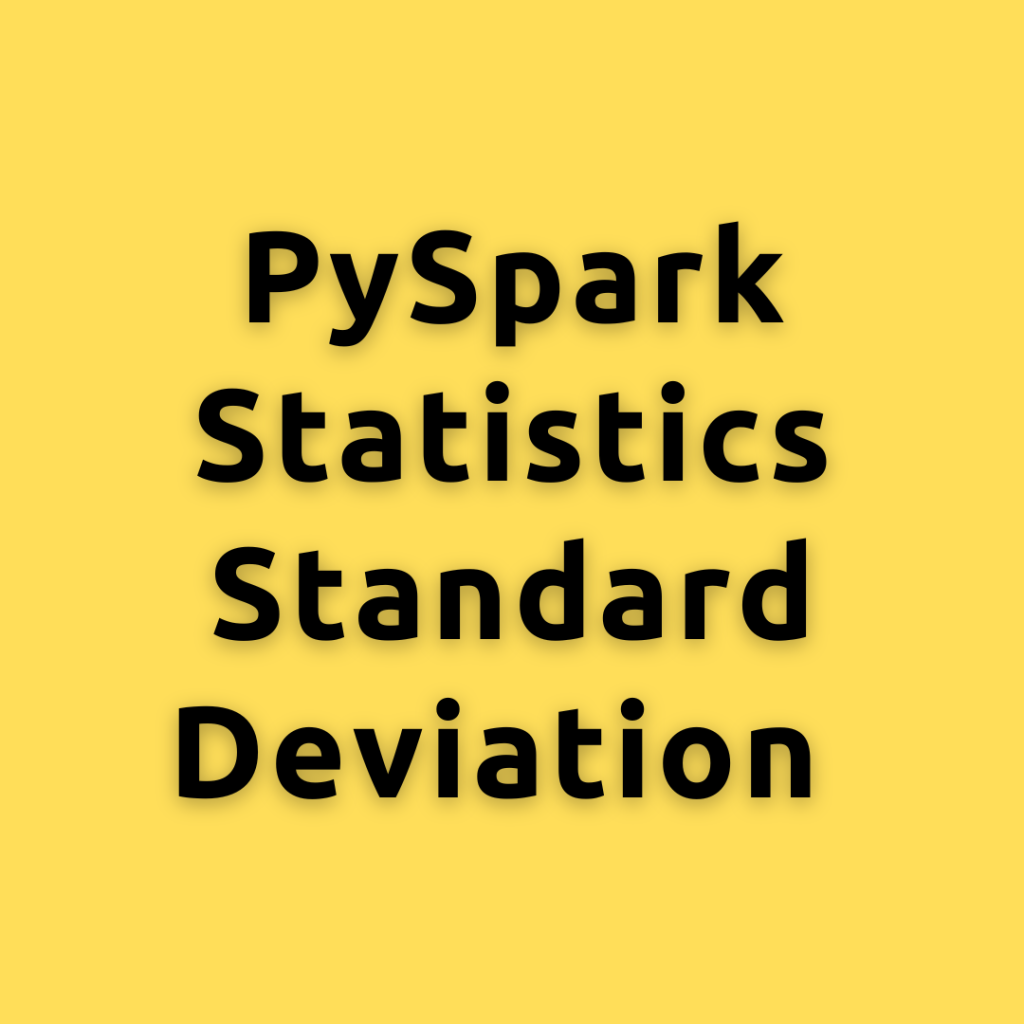## PySpark Statistics Standard Deviation – Calculating the Standard Deviation in PySpark a Comprehensive Guide for Everyone

Lets dive into the concept of Standard Deviation, its importance in statistics and machine learning, and explore different ways to calculate it using PySpark How to Calcualte Standard Deviation? Standard Deviation is a measure that quantifies the amount of variation or dispersion in a set of data values. It helps in understanding how far individual …